# Difference between revisions of "Effect of sales tax on market price and quantity traded"

This article discusses the effect of a sales tax on the market price and equilibrium quantity traded for a good.

## Assumptions

Prior to beginning the analysis, we note the following:

1. A sales tax may be revenue-proportional (proportional to the price of the trade) or quantity-proportional (proportional to the quantity being traded). The quantitative analysis differs somewhat in both these cases. However, the qualitative analysis largely does not.
2. In this article, we largely focus on the effect of the introduction of a sales tax, by performing comparative statics between a world without sales tax and a world with sales tax. Much of this analysis can also be applied to increases in sales tax. Conversely, a decrease in, or elimination of, a sales tax should have the opposite effect.
3. For the most part, we focus on short run effects. In particular, this means that we assume the law of demand and law of supply.
4. We assume away the costs of compliance with the tax laws, and do not deal with issues of tax evasion.
5. For the most part, we assume competitive markets (though we also discuss other cases). Hence, the law of one price is assumed to hold, so that we can talk of the market price.

## What we are interested in tracking

In the world with no tax, there are two measures of interest:

In a word with tax, we are interested in tracking three measures:

• The pre-tax market price, i.e., the effective price that the seller gets to keep. This is to be compared to the market price in a world without sales tax.
• The post-tax market price, i.e., the effective price that the buyer pays. This is obtained by adding the sales tax to the pre-tax market price. This is to be compared to the market price in a world without sales tax.
• The equilibrium quantity traded. This is to be compared to the equilibrium quantity traded in a world without sales tax.

## Summary of several cases

Case in question Conclusion about pre-tax market price(s) (relative to market price in a world without the tax) Conclusion about post-tax market price(s) (relative to market price in a world without the tax) Conclusion about equilibrium quantity (or quantities) traded (relative to a world without the tax)
Single good provided in a perfectly competitive market falls rises falls
Single good provided by a monopoly firm indeterminate rises falls
Two partial substitute goods each sold in perfectly competitive markets falls for at least one good rises for both goods falls for at least one good
Two complementary goods each sold in perfectly competitive markets falls for both goods rises for at least one good falls for both goods

## Relationship with other analyses

### Effect of subsidies

Subsidies are taxes in negative, so their effects on market prices and quantity traded are the exact negatives of the effects of taxes.

However, their effects on economic surplus are often in the same direction as that of taxes: negative.

## Effect of sales tax on a single good with a competitive market

We consider comparative statics between two situations:

• A world where there are no sales taxes
• A world where sales taxes are introduced on a single good (or class of goods) for which we are drawing the supply and demand curves.

Note that the same analysis also works for comparative statics where we change the sales tax on only one class of goods.

### Analytical tools

There are three kinds of diagrams that we draw to study the situation:

1. Consider the world without sales tax. We can draw the usual supply and demand curves and do the usual analysis to find the market price and equilibrium quantity traded.
2. Consider the world with sales tax. In this world, consider supply and demand curves drawn with respect to pre-tax prices.
3. Consider the world with sales tax. In this world, consider supply and demand curves with respect to post-tax prices.

### Analysis with pre-tax prices

We want to perform comparative statics between the world without sales tax and the world with sales tax. We consider the supply and demand curves for the latter in terms of the pre-tax prices.

Supply curve: The supply curve using pre-tax prices is expected to remain the same as the supply curve in a world without taxes, because the price that the seller sees is the pre-tax price.

Demand curve: The demand curve changes. In general, it moves downward. Assuming the law of demand, that is the same as moving inward, i.e., a contraction of the demand curve. Arithmetically, the new demand curve is related to the old demand curve as follows:

Case What happens to the demand curve algebraically Pictorial depiction
price-propotional sales tax For a revenue-proportional sales tax with a factor of$x$, the new demand function at a price of$p$ equals the old demand function at a price of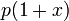$p(1 + x)$. The reason is that, as far as buyers are concerned, the effective price is$p(1 + x)$. The upshot is that the demand curve shrinks downward by a factor of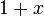$1 + x$. An example picture is below, comparing the no-tax demand curve (solid blue) with the pre-tax demand curve for a 50% sales tax (dashed purple). The original curve shrinks downward to 2/3 of its original level.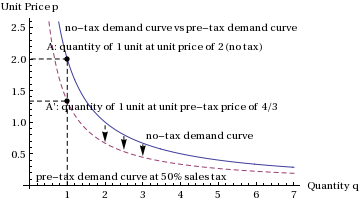quantity-proportional sales tax For a quantity-proportional sales tax with a tax of$b$ per unit quantity, the new demand function at a price of$p$ is the old demand function at a price of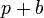$p + b$. The reason is that, as far as buyers are concerned, the effective price is$p + b$. The upshot is that the demand curve shifts downward by a vertical distance of$b$. An example picture is below, comparing the no-tax demand curve (solid blue) with the pre-tax demand curve for a 0.4 price units/unit quanity sales tax (dashed purple). The original curve shrinks downward by 0.4 price units from its original level.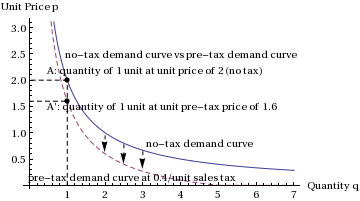The upshot is that the supply curve remains the same and the demand curve moves inward. Thus, as with the general analysis of comparative statics for demand and supply, we obtain that:

• The market price drops. In other words, the new pre-tax market price is lower than the market price in the world without taxes.
• The equilibrium quantity traded falls.

The general picture of what happens, showing the contraction of demand curve and consequent move to a lower equilibrium price and lower quantity traded, is below.

### Analysis with post-tax prices

We want to perform comparative statics between the world without sales tax and the world with sales tax. We consider the supply and demand curves for the latter in terms of the post-tax prices.

Demand curve: The demand curve using post-tax prices is expected to remain the same as the demand curve in a world without taxes, because the price that the buyer sees is the post-tax price.

Supply curve: The supply curve changes. In general, it moves upward. Assuming the law of supply, that is the same as moving inward, i.e., a contraction of the supply curve. Arithmetically, the new supply curve is related to the old supply curve as follows:

• For a revenue-proportional sales tax with a factor of$x$, the new supply function at a price of$p$ equals the old supply function at a price of$p/(1 + x)$. The reason is that, as far as sellers are concerned, the effective price is the pre-tax price$p/(1 + x)$. The upshot is that the supply curve moves upward by a factor of$1 + x$.
• For a quantity-proportional sales tax with a tax of$b$ per unit quantity, the new supply function at a price of$p$ is the old supply function at a price of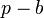$p - b$. The reason is that, as far as sellers are concerned, the effective price is$p - b$. The upshot is that the supply curve shifts upward by a vertical distance of$b$.

The upshot is that the supply curve contracts and the demand curve remains the same. Thus, as with the general analysis of comparative statics for demand and supply, we obtain that:

• The market price rises. In other words, the new post-tax market price is higher than the market price in the world without taxes.
• The equilibrium quantity traded falls.

### Combined analysis and conclusions

Combining both these analyses, we obtain the three conclusions:

• The pre-tax market price is lower than the market price in a world without the tax (this can be seen via the comparative statics between the no-tax and the pre-tax curves)
• The post-tax market price is higher than the market price in a world without the tax (this can be seen via the comparative statics between the no-tax and the post-tax curves)
• The equilibrium quantity traded is less than the equilibrium quantity traded in a world without the tax (this can be seen using either of the two comparative statics methods employed above)

### Extreme cases of elastic and inelastic supply and demand

We consider some extreme cases. The first row describes the standard case, and subsequent rows describe extreme cases:

Assumption for price-elasticity of demand Assumption for price-elasticity of supply Conclusion about pre-tax market price (relative to market price in a world without the tax) Conclusion about post-tax market price (relative to market price in a world without the tax) Conclusion about equilibrium quantity traded (relative to a world without the tax)
negative (satisfies the law of demand) positive (satisfies the law of supply) falls rises falls
infinite, i.e., a horizontal demand curve (e.g., when the good has a perfect substitute) positive (satisfies the law of supply) falls stays the same falls
zero, i.e., a vertical demand curve. We also say that the demand is perfectly price-inelastic positive (satisfies the law of supply) stays the same rises stays the same
negative (satisfies the law of demand) infinite, i.e., a horizontal supply curve, e.g., a constant cost industry. Alternatively, this also applies if the jurisdiction where sales tax is imposed is a small subjurisdiction of the economy and the pre-tax prices of the goods are determined by the world economy. stays the same rises falls
negative (satisfies the law of demand) zero, i.e., a vertical supply curve. We say that the supply is perfectly price-inelastic falls stays the same stays the same

### How price-elasticity affects the nature of the effect of sales tax

The extent to which the sales tax affects the pre-tax price, post-tax price, and equilibrium quantity traded depends upon the price-elasticity of demand and price-elasticity of supply. The following are two general principles:

• Between demand and supply, the relatively more price-inelastic side absorbs more of the price burden of the sales tax. In other words, if demand is more inelastic, then the effect of the sales tax is largely seen in terms of an increase in the post-tax price. If supply is more inelastic, then the effect of the sales tax is largely seen in terms of a decrease in the pre-tax price. This is in keeping with the general principle attributed to Ricardo that rents are captured by the most inelastic side. Here, the rents are reversed in sign, but the principle stays the same.
• In general, the extent to which the equlibrium quantity traded is affected is negatively related to the price-elasticities of both demand and supply. In other words, if we reduce the price-elasticity of either demand or supply, the sensitivity of the equilibrium quantity traded to the sales tax reduces. In particular, if either demand or supply is perfectly price-inelastic, the equilibrium quantity traded is independent of the sales tax.

## Effect of sales tax on a single good with a monopolist-controlled market

This section assumes familiarity with the key conclusions of determination of price and quantity supplied by monopolistic firm in the short run.

We consider comparative statics between two situations:

• A world where there are no sales taxes
• A world where sales taxes are introduced on a single good (or class of goods) that is supplied by a single seller (the monopolist) that seeks to maximizing profit.

The marginal revenue from the sale of a good equals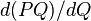$d(PQ)/dQ$, as$PQ$ is revenue and we are interested in the rate of change of revenue as quantity changes. Using the Product Rule of calculus, we find that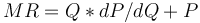$MR = Q*dP/dQ + P$. As explained previously, we expect that dP/dQ is negative in accordance with the law of demand. As such, marginal revenue is less than the price paid, and it may even be zero or negative.

Unlike the perfectly competitive market case discussed previously, we do not perform two separate analyses (pre-tax and post-tax). Rather, we consider various pre-tax and post-tax curves together. The reason is that a "post-tax supply curve" does not make sense in the monopoly case. Also, the analysis is complicated enough as it is, so creating two versions of it isn't worth it!

### Key curves under consideration

We want to perform comparative statics between the world without sales tax and the world with sales tax. We consider the marginal cost, demand, and marginal revenue curves for the latter in terms of the pre-tax prices.

Pre-tax marginal cost curve: The marginal cost curve using pre-tax prices is expected to remain the same as the marginal cost curve in a world without taxes, because the price that the seller sees is the pre-tax price.

Pre-tax demand curve: The pre-tax demand curve changes relative to what it is in a world without taxes. In general, it moves downward. Assuming the law of demand, that is the same as moving inward, i.e., a contraction of the demand curve. Arithmetically, the new demand curve is related to the old demand curve either through proportional shrinkage toward the quantity axis (for a revenue-proportional sales tax) or through a downward translation toward the quantity by the amount of the tax on unit quantity. For more, see the analysis of pre-tax prices section for the perfectly competitive case.

Pre-tax marginal revenue curve: The pre-tax marginal revenue curve changes relative to what it is in a world without taxes. Qualitatively, the way it changes is the same as the way the demand curve changes. For a revenue-proportional sales tax, it shrinks proportionally toward the quantity axis. For a quantity-proportional sales tax, it is translated downward. This can be inferred easily from the definition of marginal revenue as$d(PQ)/dQ$, and the way derivatives interact with addition and scalar multiplication (specifically, the fact that differentiation is linear).

Post-tax demand curve (demand curve as experienced by buyers): This remains the same as it would be in a world without taxes.

The upshot: the pre-tax marginal cost curve remains unchanged and the pre-tax marginal revenue curve shrinks downward. We now discuss the implications this has for our conclusions around pre-tax price and quantity traded. Prima facie, this looks a lot like the analysis so far with pre-tax prices for a perfectly competitive market, with marginal revenue curve replacing demand curve and marginal cost curve replacing supply curve. However, there are three subtleties that complicate the picture

1. The (price, quantity) pair at which trades actually occur is not the point of intersection of the marginal cost and marginal revenue curves. Rather, the quantity is determined by the point of intersection, but the price is determined by looking at the demand curve. This distinction alone causes some of our conclusions to break down.
2. Unlike the demand curve in a perfectly competitive market, the marginal revenue curve is not necessarily downward-sloping. In fact, it need not even always be above the quantity axis! It can have multiple local maxima and local minima. The key distinction is that marginal revenue is affected by a combination of two factors pulling in opposite direction: expansion of the market, and reduction in the price that can be charged to buyers with the highest reservation prices.
3. Unlike the supply curve in a perfectly competitive market, the marginal cost curve is not necessarily upward-sloping. It can have multiple local maxima and local minima. The key distinction with the competitive case that the supply curve for a seller in a competitive market is not the entire marginal cost curve but only a part of it that slopes upward. For more, see determination of quantity supplied by firm in perfectly competitive market in the short run.

There are a wide range of cases where we can make assumptions to eliminate problems (2) and (3). However, problem (1) remains.

### The easy case: marginal cost curve increasing everywhere, marginal revenue curve decreasing everywhere

This is the easy case where, of the three problems mentioned, only Problem (1) applies.

In the case that the marginal cost curve is increasing throughout the relevant range (outside of which no optima can possibly exist) and the marginal revenue curve is decreasing throughout the relevant range, our pictorial analysis works as in the perfectly competitive case. In particular:

• Since the marginal revenue curve has shrunk downward and leftward, the new point of intersection of the curves has a lower price and lower quantity traded. Thus, the quantity traded is lower than in a world without sales tax. Also, the pre-tax marginal cost experienced by the seller is lower than in a world without sales tax.
• The post-tax price charged to buyers is higher than what it would be in a world without sales tax. This is because the quantity traded is lower, so referencing the post-tax demand curve (which is the same as the original demand curve) we get a higher price.
• However, even in this case, the effect on the pre-tax price is ambiguous. This is because there are two competing effects. On the one hand, the quantity traded is lower, causing the maximum price chargeable to increase. On the other hand, the pre-tax demand curve itself has shrunk, causing the price chargeable to decrease.

### The more general case

In the more general case, Problems (2) and (3) can also arise. Specifically, the marginal revenue curve can even go below the quantity axis, and the marginal cost curve can be non-monotonic. In such a situation, we can have multiple locally optimal configurations. When a sales tax is imposed, a monopolist may switch from one optimum to another. An example is in the box.

To illustrate how this latter possibility could happen, imagine that one buyer of the good is willing to pay \$3000 for a single unit while all subsequent buyers are willing to pay \$2 per unit (up to the 10,000th unit). Imagine also that the marginal cost of producing the first unit is \$500, while the marginal cost of producing all units thereafter is \$1. The marginal revenue for the first unit is \$3000, for the second unit is -\$2996 (as the seller would get \$2 for each of the two units sold but would effectively lose the ability to charge \$3000 for the first unit), and for all units thereafter is \$2. With no tax in place, the monopolist would choose to sell 10,000 units for \$2 each, making a profit of \$9501 (\$20,000 - \$9,999 - \$500) rather than only selling one unit for \$3000 (which would only make a profit of \$2500). Now suppose a 300% sales tax is imposed. This effectively reduces all marginal revenue figures by 3/4ths. Selling units to customers only willing to pay \$2 per unit would no longer be possible, as the pre-tax price could be \$0.50 at maximum, and the marginal cost of producing units is \$1. However, the buyer willing to pay \$3000 could pay a pre-tax price of \$750 and the monopolist could make a profit of \$250 selling to that buyer alone. So the result of the sales tax in this (unusual) case would be to raise the pre-tax price of the unit from \$2 to \$750.

However, it turns out that any such optimum-switching must be in the direction of a market shrinkage. In other words, even if we see optimum-switching, where a monopolist switches strategy, it will still be true that:

• The quantity traded decreases relative to a world without sales tax.
• The post-tax market price increases relative to a world without sales tax.

The justification is a little complicated, since we can no longer rely on visual reasoning but must perform careful comparative statics.

#### What's common between revenue-proportional and quantity-proportional sales taxes

To understand why the conclusion holds both for revenue-proportional and for quantity-proportional sales taxes, we note the following. The comparisons are between different choices of (price, quantity), holding the sales tax level constant.

• For a revenue-proportional sales tax, the larger the total post-tax revenue, the larger the absolute tax burden.
• For a quantity-proportional sales tax, the larger the total quantity traded, the larger the absolute tax burden.

#### The explanation for why quantity traded must decrease and post-tax market price must increase under the introduction of a sales tax

Denote by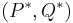$(P^*, Q^*)$ the price and quantity chosen by the monopolist in a world without tax. Suppose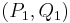$(P_1, Q_1)$ is a (price, quantity) pair on the post-tax demand curve with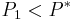$P_1 < P^*$ (and therefore$Q_1 > Q^*$). We will show that the profit from the latter configuration under sales tax is less than the profit under the sales tax from the original optimal configuration$(P^*, Q^*)$.

First, we note that by the definition of optimality:

Profit without sales tax under$(P^*, Q^*)$$\ge$ Profit without sales tax under$(P_1, Q_1)$

We also have:

Profit with sales tax under$(P^*, Q^*)$ = Profit without sales tax under$(P^*, Q^*)$ - Absolute tax burden under$(P^*, Q^*)$

Profit with sales tax under$(P_1, Q_1)$ = Profit without sales tax under$(P_1, Q_1)$ - Absolute tax burden under$(P_1, Q_1)$

Also, as discussed in the preceding subsection, it must be the case that:

Absolute tax burden under$(P_1, Q_1)$ > Absolute tax burden under$(P^*, Q^*)$

Combining these inequalities, we obtain that:

Profit with sales tax under$(P^*, Q^*)$ > Profit with sales tax under$(P_1, Q_1)$

This provides the desired conclusion.

### Conclusion

• The pre-tax price (as received by the monopolist) may increase or decrease. Note that this indeterminacy is present even in the case of an increasing marginal cost curve and decreasing marginal revenue curve. The expected proof breaks down due to the fact that the pre-tax price itself is not obtained by intersecting the two curves.
• The post-tax price (as seen by buyers) must increase. This observation depends on the law of demand, but does not make any additional assumptions about the marginal cost and marginal revenue curve.
• The quantity traded must decrease. This observation depends on the law of demand, but does not make any additional assumptions about the marginal cost and marginal revenue curve.
• In the special case that we are dealing with an increasing marginal cost curve and a decreasing marginal revenue curve, we can conclude that the marginal cost seen by the seller at the quantity that the seller produces goes down relative to what it was in a world without sales tax.

## Effect of sales tax that also affects complementary and substitute goods

The analysis of the preceding section was based on the assumption that the sales tax is levied only on that particular good for which the analysis is being performed. This assumption is necessary to ensure that the other determinants of demand and determinants of supply are unaffected.

However, in real world situations, sales taxes are levied on large classes of goods, and changes to sales taxes are made simultaneously on large classes of goods. In particular, the sales tax may also affect the market prices of complementary goods and substitute goods. This means that we either need a more complicated partial equilibrium analysis (that somehow accounts for the prices of all the complementary and substitute goods) or an even more complicated general equilibrium analysis. This is extremely tricky. We consider some special cases to illustrate the kinds of effects that may be operational.

### Sales tax on two mutually substitute goods

Consider two goods$A,B$ that are partial substitutes for each other as far as buyers are concerned. Starting with a world with no sales tax, a sales tax is then levied on both$A$ and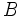$B$. Also assume that the suppliers of$A$ and$B$ are disjoint, so there is no supply-side substitution. We can draw the following conclusions:

1. The post-tax market prices for both$A$ and$B$ are higher than the market price in a world without taxes.
2. The pre-tax market price for at least one of$A$ and$B$ must fall (or stay the same) relative to its market price. In other words, it cannot happen that both pre-tax market prices are higher than the respective market prices in a world without taxes.
3. The equilibrium quantity traded for at least one of$A$ and$B$ must fall (or stay the same) relative to the original equilibrium quantity traded. In other words, it cannot happen that the quantity traded for both goods rises relative to the world without taxes.

The justification of these using a combined partial equilibrium analysis is hard, but we can try to do a seriatim analysis of the two goods:

• In the first approximation, ignore the substitution effects entirely. Then, the analysis proceeds as in the preceding section, so we expect that for both$A$ and$B$, in the first approximation, that the pre-tax price is lower than the original market price, the post-tax price is higher than the original market price, and the equilibrium quantity traded is lower than the original.
• We now consider the substitution effects. Since the post-tax price for$B$ has risen, this is a change to one of the determinants of demand for$A$, and the effect of the change is an expansion in the demand curve for$A$. This expansion applies to both the pre-tax and post-tax demand curves. The upshot is that the pre-tax price goes up and the post-tax price goes up too, while the equilibrium quantity traded rises.
• The combined effect on the pre-tax price is ambiguous (in the first approximation, it goes down, but the substitution effect pushes it back up, and it is unclear which effect dominates). The combined effect on the equilibrium quantity traded is also ambiguous. However, the combined effect on the post-tax price is unambiguous: both reasons cause it to go up.
• Of course, these are just two approximations. We can continue the process ad infinitum -- we can use the changes to the price of$A$ to shift the demand curve for$B$ via the substitution effect, and then again back to$A$, and so on. The hope is that the process will eventually converge. To avoid this kind of infinite process, a combined partial equilibrium analysis is useful.

The crude seriatim analysis does show that the post-tax price goes up. However, it does not clearly show the conclusions mentioned about pre-tax price and quantity traded. Those conclusions can be better derived by thinking of the combined market for both goods and applying a single analysis to that.

### Sales tax on two mutually complementary goods

Consider two goods$A,B$ that are complements for each other as far as buyers are concerned. Starting with a world with no sales tax, a sales tax is then levied on both$A$ and$B$. Also assume that the suppliers of$A$ and$B$ are disjoint, so there is no supply-side complementation or substitution. We can draw the following conclusions:

1. The pre-tax market prices for both$A$ and$B$ are lower than the market price in a world without taxes.
2. The post-tax market price for at least one of$A$ and$B$ must rise (or stay the same) relative to its market price. In other words, it cannot happen that both post-tax market prices are lower than the respective market prices in a world without taxes.
3. The equilibrium quantity traded for both$A$ and$B$ must fall (or stay the same) relative to the original equilibrium quantity traded.

The justification of these using a combined partial equilibrium analysis is hard, but we can try to do a seriatim analysis of the two goods, similar to that done for substitution effects.

• In the first approximation, ignore the complementarity effects entirely. Then, the analysis proceeds as in the preceding section, so we expect that for both$A$ and$B$, in the first approximation, that the pre-tax price is lower than the original market price, the post-tax price is higher than the original market price, and the equilibrium quantity traded is lower than the original.
• We now consider the complementarity effects. Since the post-tax price for$B$ has risen, this is a change to one of the determinants of demand for$A$, and the effect of the change is an contraction in the demand curve for$A$. This contraction applies to both the pre-tax and post-tax demand curves. The upshot is that the pre-tax price goes down and the post-tax price goes down too, while the equilibrium quantity traded falls.
• The combined effect on the post-tax price is ambiguous (in the first approximation, it goes up, but the complementarity effect pushes it back down, and it is unclear which effect dominates). The combined effects on the pre-tax price and equilibrium quantity traded are unambiguous, however: both reasons cause them to go down.
• Of course, these are just two approximations. We can continue the process ad infinitum -- we can use the changes to the price of$A$ to shift the demand curve for$B$ via the complementarity effect, and then again back to$A$, and so on. The hope is that the process will eventually converge. To avoid this kind of infinite process, a combined partial equilibrium analysis is useful.

The crude seriatim analysis does show that the pre-tax price and quantity traded both go down. However, it does not clearly show the conclusions mentioned about the post-tax price. Those conclusions can be better derived by thinking of the combined market for both goods and applying a single analysis to that.